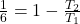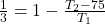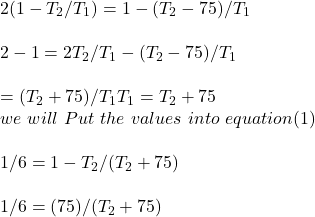## The efficiency of a carnot cycle is 1/6. If on reducing the temperature of the sink 75 degree Celsius, the efficiency becomes 1/3, determine

Question

The efficiency of a carnot cycle is 1/6. If on reducing the temperature of the sink 75 degree Celsius, the efficiency becomes 1/3, determine the initial and final temperature between which the cycle is working.

in progress 0
5 months 2021-08-15T15:23:47+00:00 1 Answers 7 views 0

## Answers ( )

375 and 450

Explanation:

The computation of the initial and the final temperature is shown below:

In condition 1:

The efficiency of a Carnot cycle isSo, the equation isFor condition 2:

Now if the temperature is reduced by 75 degrees So, the efficiency isTherefore the next equation isNow solve both the equations

solve equations (1) and (2)T_2 + 450 = 75

T_2 = 375

Now put the T_2 value in any of the above equation

i.e

T_1 = T_2 + 75

T_1 = 375 + 75

= 450